### Total 104 Videos found in Category "Chemistry"

Page: 1 2 3
Title
 1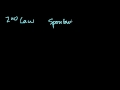A look at a seductive but wrong Gibbs/Spontaneity Proof 2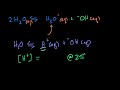Acid Base Introduction 3Acid Base Titration 4Another mass composition problem 5Another Stoichiometry Example in a Solution 6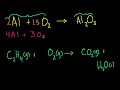Balancing Chemical Equations 7Boiling Point Elevation and Freezing Point Supression 8Buffers and Hendersen-Hasselbalch 9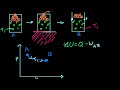Carnot Cycle and Carnot Engine 10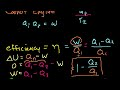Carnot Efficiency 2: Reversing the Cycle 11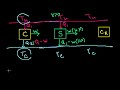Carnot Efficiency 3: Proving that it is the most efficient 12Change of State Example 13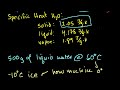Chilling Water Problem 14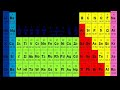Conjugate Acids and Bases 15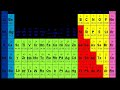Covalent Networks, Metallic, and Ionic Crystals 16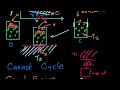Efficiency of a Carnot Engine 17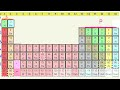Electron Configurations 18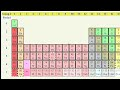Electron Configurations 2 19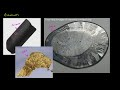Elements and Atoms 20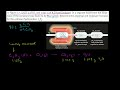Empirical and Molecular Formulas from Stoichiometry 21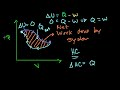Enthalpy 22Entropy Intuition 23Example of Finding Reactant Empirical Formula 24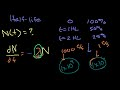Exponential Decay Formula Proof (can skip, involves Calculus) 25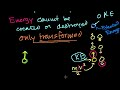First Law of Thermodynamics/ Internal Energy 26Formula from Mass Composition 27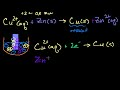Galvanic Cells 28Gibbs Free Energy and Spontaneity 29Gibbs Free Energy Example 30Groups of the Periodic Table 31Half Equivalence Point 32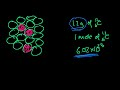Half-Life 33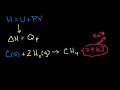Heat of Formation 34Hess Law and Reaction Enthalpy Change 35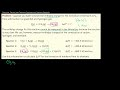Hess Law Example 36Heterogenous Equilibrium 37Hydrogen Peroxide Correction 38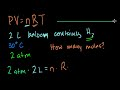Ideal Gas Equation Example 1 39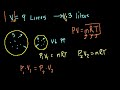Ideal Gas Equation Example 2 40Ideal Gas Equation Example 3 41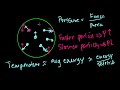Ideal Gas Equation: PV=nRT 42Ideal Gas Example 4 43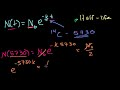Introduction to Exponential Decay 44Introduction to Kinetics 45Introduction to Oxidation States 46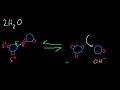Introduction to pH, pOH, and pKw 47Introduction to the atom 48Ionic, Covalent, and Metallic Bonds 49Keq derivation intuition (can skip; bit mathy) 50Keq Intuition (mathy and not necessary to progress)

Say and share some thing about these videos...